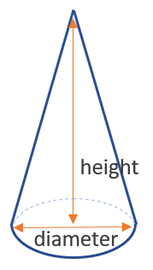# Volume of a Cone Calculator

Use this cone volume calculator to easily calculate the volume of a cone from its base radius and height in any metric: mm, cm, meters, km, inches, feet, yards, miles...

### Calculation results

47.123890 cm3 Cone volume
Share calculator:

Embed this tool:
get code

## Volume of a cone formula

The formula for the volume of a cone is (height x π x (diameter / 2)2) / 3, where (diameter / 2) is the radius of the base (d = 2 x r), so another way to write it is (height x π x radius2) / 3, as seen in the figure below:Despite the relative complexity of the body, you only need two measurements to calculate a cone's volume: its height and the diameter of its base, or equivalently - its radius. π is the unitless mathematical constant equal to ~3.149159.

## How to calculate the volume of a cone?

First, take the measurement of the diameter (widest measurement you can take of the base), then measure or estimate the height. If you already have plans or schematics, just get the lengths from there. Convert the length units to the same base, e.g. inches or centimeters, then follow the formula above or use our online volume of a cone calculator. The output is always in cubic units, e.g. cubic inches, cubic feet, cubic yards, cubic mm, cubic cm, cubic meters, and so on.

## Example: find the volume of a cone

Applying the cone volume equations is straightforward provided the cone's height is known and one of the following is also given: the radius, the diameter, or the area of its base. For example, if the height and area are given to be 2 feet and 15 square feet, the cone volume is then simply the result of multiplying the two and dividing by three: 2 x 15 / 3 = 30 / 3 = 10 cubic feet.

Using the second equation is appropriate if the area is unknown, but the radius can be measured or is given. Calculating the volume requires just one extra step. For example, if the height is 20 inches and the radius is 4 inches, the area can be calculated by 3.14159 x 42 = 3.14159 x 16 = 50.265. The volume is then 20 x 50.26544 / 3 = 1005.31 / 3 = 335.1 cu in. A higher level of precision for π will result in more accurate results which is why using a cone volume calculator is preferred.

## Practical applications

The cone is not as popular as some other bodies in construction and engineering, but it has its uses. One of the first examples you can think of are traffic cones, which might be filled with water or other material to keep them in place, especially in windy locations.

#### Cite this calculator & page

If you'd like to cite this online calculator resource and information as provided on the page, you can use the following citation:
Georgiev G.Z., "Volume of a Cone Calculator", [online] Available at: https://www.gigacalculator.com/calculators/volume-of-cone-calculator.php URL [Accessed Date: 28 Mar, 2023].